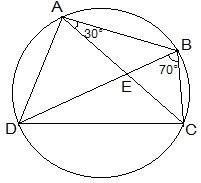Guru

# ABCD is a cyclic quadrilateral whose diagonals intersect at a point E. If ∠ DBC = 70°, ∠ BAC is 30°, find ∠ BCD. Further, if AB = BC, find ∠ ECD. Q.6

• 0

Please give me the best way for solving the problem of class 9th ncert math of Circles chapter of math of class 9th of exercise 10.5of question no 6 what is the tricky way for solving this question ABCD is a cyclic quadrilateral whose diagonals intersect at a point E. If ∠ DBC = 70°, ∠ BAC is 30°, find ∠ BCD. Further, if AB = BC, find ∠ ECD

Share

1. Consider the following diagram.Consider the chord CD,

We know that angles in the same segment are equal.

So, ∠ CBD = ∠ CAD

Now, ∠ BAD will be equal to the sum of angles BAC and CAD.

= 30°+70°

We know that the opposite angles of a cyclic quadrilateral sums up to 180 degrees.

So,

It is known that ∠ BAD = 100°

So, ∠ BCD = 80°

Now consider the ΔABC.

Here, it is given that AB = BC

Also, ∠ BCA = ∠ CAB (They are the angles opposite to equal sides of a triangle)

∠ BCA = 30°

also, ∠ BCD = 80°

∠ BCA +∠ ACD = 80°

Thus, ∠ ACD = 50° and ∠ ECD = 50°

• 0